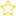See the live dashboard for CensusAtSchool 2023

More generally, when working with a discrete random variable, X, (e.g. Poisson or Binomial) does the word ‘between’ mean include or exclude the endpoints?

“What is the probability that X is between 3 and 7” is ambiguous with respect to the inclusion or exclusion of the endpoints when X is a discrete random variable.

There is NO consensus whether ‘between’ means include or exclude the endpoints. If you use the word ‘between’ with a discrete random variable then you have to explicitly tell the students whether you are including or excluding the endpoints.

Pr(3< X ≤ 7) can be clearly and unambiguously written in words in a number of ways such as:

• What is the probability that X is between 3 and 7, not including 3 but including 7?
• What is the probability that X is bigger than 3 but no bigger than 7?
• What is the probability that X is at least 4 but at most 7?
• What is the probability that X is greater than 3 but less than or equal to 7?

and so on.

Of course if X is a continuous random variable (Normal, uniform continuous or the triangular continuous) then the inclusion or exclusion of the endpoints is irrelevant and you may freely use the word ‘between’ without clarifying whether the endpoints are included or excluded.

One more thing: don’t believe everything you read on Wolfram – it’s on a level similar to Wikipedia.

• Keywords: Binomial, FAQ, Poisson, Probability, Probability distributions
• (Last updated: 03/05/18. Added: 03/05/18)
• ## Rate this resource:(No ratings yet)